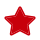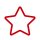Updated at: 2022-08-03 GMT+08:00

# PG_STATISTIC_EXT

PG_STATISTIC_EXT records the extended statistics of tables in a database, such as statistics of multiple columns. Statistics of expressions will be supported later. You can specify the extended statistics to be collected. It is accessible only to users with system administrator rights.

Table 1 PG_STATISTIC_EXT columns

Parameter

Type

Description

starelid

oid

Table or index which the described column belongs to

starelkind

"char"

Type of an object

stainherit

boolean

Whether to collect statistics for objects that have inheritance relationship

stanullfrac

real

Percentage of column entries that are null

stawidth

integer

Average stored width, in bytes, of non-null entries

real

Number of distinct, not-null data values in the column for all DNs

• A value greater than zero is the actual number of distinct values.
• A value less than zero is the negative of a multiplier for the number of rows in the table. (For example, stadistinct=-0.5 indicates that values in a column appear twice on average.)
• 0 indicates that the number of distinct values is unknown.

real

Number of unique non-null data values in the dn1 column

• A value greater than zero is the actual number of distinct values.
• A value less than zero is the negative of a multiplier for the number of rows in the table. (For example, stadistinct=-0.5 indicates that values in a column appear twice on average.)
• 0 indicates that the number of distinct values is unknown.

stakindN

smallint

Code number stating that the type of statistics is stored in Slot N of the pg_statistic row.

Value range: 1 to 5

staopN

oid

Operator used to generate the statistics stored in Slot N. For example, a histogram slot shows the < operator that defines the sort order of the data.

Value range: 1 to 5

stakey

int2vector

Array of a column ID

stanumbersN

real[]

Numerical statistics of the appropriate type for Slot N. The value is null if the slot kind does not involve numerical values.

Value range: 1 to 5

stavaluesN

anyarray

Column data values of the appropriate type for Slot N. The value is null if the slot type does not store any data values. Each array's element values are actually of the specific column's data type so there is no way to define these columns' type more specifically than anyarray.

Value range: 1 to 5

staexprs

pg_node_tree

Expression corresponding to the extended statistics information.